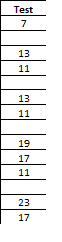Select a Column of Non-Sequential DataIn one of my posts on using VBA to select a column of data, I received a comment asking if the macro could be modified to select data like the screen shot you see to the left.

This data is non-sequential and consequently the CurrentRegion property won’t work. The way around this is to select the very last row in the same column, then shoot up (Ctrl + Up Arrow) to find the last data cell.

Once you know the where the column heading and last data cell is in the current column, the range can then be selected.

The following macro will select the column of data if you start with the active cell at the column heading.

Sub SelectOneColumnData()
'''''''''''''''''''''''''''''''''''''''''''''''''''''''''''''''
' This routine will select a non-continuous column of data
' when active cell is located in the column heading.
'''''''''''''''''''''''''''''''''''''''''''''''''''''''''''''''
Dim ac As Range
Dim lRow As Long
Dim lc As Range
Dim col As Integer
Dim cr As Range

Set ac = ActiveCell
col = ac.Column
lRow = ActiveSheet.Rows.Count
Set lc = Cells(lRow, col)

' Find the bottom of the range then re-set the last cell range
Set lc = lc.End(xlUp)
lRow = lc.Row

' Set the current range from the active cell to the last row
' in the column with data
Set cr = ac.Offset(1, 0).Resize(lc.Row - ac.Row, 1)
cr.Select
End Sub